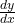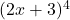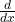## find the derivatives of the following y=(2x+3)⁴ ​

Question

find the derivatives of the following y=(2x+3)⁴ ​

in progress 0
2 months 2021-08-04T18:45:33+00:00 1 Answers 4 views 0= 8(2x + 3)³

Step-by-step explanation:

Using the function of a function rule

Given

y = f(g(x)) , then= f'(g(x)) × g'(x)

Given

y =, then= 4(2x + 3)³ ×(2x + 3)

= 4(2x + 3)³ × 2

= 8(2x + 3)³